Next: Term frequency and weighting Up: Parametric and zone indexes Previous: Learning weights   Contents   Index

## The optimal weight g

We begin by noting that for any training example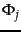for which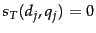and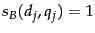, the score computed by Equation 14 is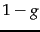. In similar fashion, we may write down the score computed by Equation 14 for the three other possible combinations of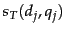and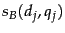; this is summarized in Figure 6.6 .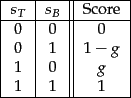Let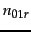(respectively,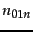) denote the number of training examples for whichandand the editorial judgment is Relevant (respectively, Non-relevant). Then the contribution to the total error in Equation 17 from training examples for whichandis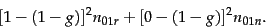(18)

By writing in similar fashion the error contributions from training examples of the other three combinations of values forand(and extending the notation in the obvious manner), the total error corresponding to Equation 17 is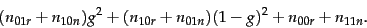(19)

By differentiating Equation 19 with respect to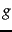and setting the result to zero, it follows that the optimal value ofis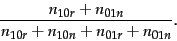(20)

Exercises.

• When using weighted zone scoring, is it necessary for all zones to use the same Boolean match function?

• In Example 6.1.1 above with weights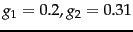and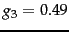, what are all the distinct score values a document may get?

• Rewrite the algorithm in Figure 6.4 to the case of more than two query terms.

• Write pseudocode for the function WeightedZone for the case of two postings lists in Figure 6.4 .

• Apply Equation 20 to the sample training set in Figure 6.5 to estimate the best value offor this sample.

• For the value ofestimated in Exercise 6.1.3, compute the weighted zone score for each (query, document) example. How do these scores relate to the relevance judgments in Figure 6.5 (quantized to 0/1)?

• Why does the expression forin (20) not involve training examples in which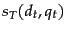and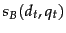have the same value?Next: Term frequency and weighting Up: Parametric and zone indexes Previous: Learning weights   Contents   Index